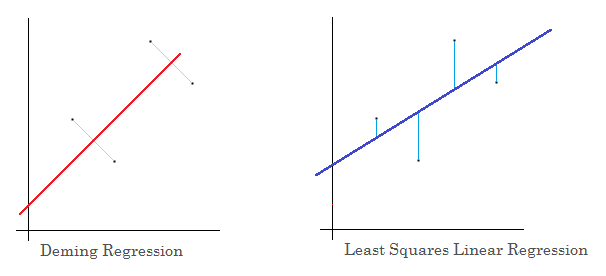# How to Minimize the Sum of Perpendicular Offsets

## Deming Linear Regression

Enter X and Y Data Pairs Below
 X Y
 X Y
 X Y

#### What is Deming Regression?

In ordinary least squares linear regression, you find the values of m and b that minimize the sum of the squared vertical distances between each point (xi, yi) and the regression line y = mx + b. That is, least squares regression determines the values of m and b that minimize the function

F(m, b) = ∑(yi - mxi - b)².

This type of regression is appropriate when you expect the variance to come solely from the measured y values, not the experimentally controlled x values. However, in many experiments, both the y and x values are observed, and neither is controlled by the person running the experiment.

In these situations, it is more appropriate to find a regression line that minimizes the sum of the squared perpendicular errors, that is, the line that minimizes the squares of the actual distances between the points and the line. This is often known as Deming Linear Regression. If the Deming regression line is y = px + q, then p and q are the values that minimize the function

G(p, q) = (1+p²)-1∑(yi - pxi - q)².

This looks very similar to the standard least squares equation F(m, b), except for the factor of (1+p²)-1. Unlike the standard least squares linear regression model, the Deming model cannot be solved with matrices. That is, the system of equations ∂G/∂p = 0 and ∂G/∂q = 0 is not linear.

Nevertheless, with a fair amount of algebra, you can find the Deming regression line by solving the equations

Ap² + Bp - A = 0
q = y - px

where

A = (∑xi)(∑yi) - n∑xiyi
B = n∑y²i - n∑x²i - (∑yi)² + (∑xi

The equation for the slope p may admit more than one solution, so you must look at a plot of the data to see which value of p matches the data trend.

#### Illustration and Example

Note the graphical difference between Deming regression and standard least squares linear regression in the figure belowSince Deming regression minimizes the sum of the squared perpendicular offsets rather than the vertical offsets, the slope and intercept of the Deming regression line is usually different from the slope and intercept of the ordinary least squares line. For instance, suppose you wish to find a line to the data set

(4, 3)   (2, 5)   (8, 7)   (6, 9)

Using the calculator above, the Deming linear regression line is

yD = x + 1.

But using an ordinary least squares regression calculator, the equation is

yLS = 0.6x + 3.

The first equation is a better fit if you treat the x and y values equally, assuming that both may have error. The second equation is a better fit if the x values are chosen by the experimenter and the y values are observed, and therefore subject to variation.